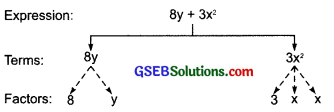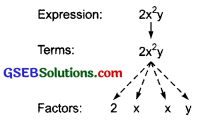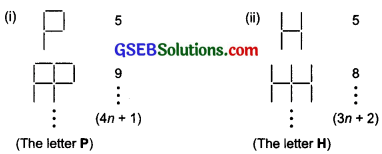# GSEB Solutions Class 7 Maths Chapter 12 Algebraic Expressions InText Questions

Gujarat Board GSEB Textbook Solutions Class 7 Maths Chapter 12 Algebraic Expressions InText Questions and Answers.

## Gujarat Board Textbook Solutions Class 7 Maths Chapter 12 Algebraic Expressions InText QuestionsTry These (Page 230)

Question 1.
Describe expressions are obtained:
(i) 7xy + 5
(ii) x²y
(iii) 4x² – 5x
Solution:
(i) 7xy + 5
To obtain this expression, we first multiply two variables x and y, i.e. x × y = xy.
Then we multiply their product by 7 to get 7xy.
Next, we add 5 to 7xy to obtain 7xy + 5.

(ii) x²y
First, x is multiplied by itself i.e., x × x = x²
Then multiply x² by y to get the required expression, i.e. x² × y = x²y

(iii) 4x² – 5x
Here, the variable x is multiplied by itself, i.e. x × x = x²
Then x² is multiplied by 4, i.e. x² x 4 = 4x²
Next, we multiply the variable x by 5, i.e. x × 5 = 5x
Now, we subtract 5x from 4x² to get 4x² – 5xTry These (Page 231)

Question 1.
What are the terms in the following expressions? Show how the terms are formed. Draw a tree diagram for each expression:
(i) 8y + 3x²
(ii) 7mn – 4
(iii) 2x²y
Solution:
(i) 8y + 3x²
Terms of 8y + 3x² are 8y and 3x²
The term 8y is formed by multiplying the variable y by 8.
The term 3x² is formed by first multiplying the variable x with itself to get x × x = x² and then multiplying x² by 3.

Tree diagram:(ii) 7mn
Terms of 7mn – 4 are 7mn and – 4.
The term 7mn formed by first multiplying variables m and n to get m x n = mn
Then multiplying mn by constant 7 to get 7 x mn = 7mn. The term – 4 is a constant.

Tree diagram:(iii) 2x²y
This expression has only one term, i.e. 2x²y. To form this term first we multiply the variable x by itself to get x × x = x².
Then the variable y is multiplied by x² to get y × x² = x²y
Next, the product x1y is multiplied by 2 to get 2 × x²y = 2x²y

Tree diagram:Question 2.
Write tree expressions each having 4 terms.
Solution:
(i) 2x3 – 4x² + 9xy + 8
(ii) 6x3 + 9y² – 3xy² – 12
(iii) 9x² – 3x + 12xy – 1Try These (Page 231)

Question 1.
Identify the coefficients of the terms of following expressions:
(i) 4x – 3y
(ii) a + b + 5
(iii) 2y + 5
(iv) 2xy
Solution:
(i) 4x – 3y
The coefficient of x in 4x is 4.
The coefficient of y in – 3y is (- 3).

(ii) a + b + 5
The coefficient of a is 1.
The coefficient of b is 1.
The coefficient of 5 is 1.

(iii) 2y + 5
The coefficient of y in 2y is 2.
The coefficient of 5 is 1.

(iv) 2xy
The coefficient of xy in 2xy is 2.
The coefficient of y in 2xy is 2x.
The coefficient of y in 2xy is 2y.

Try These (Page 233)

Question 1.
Group the like terms together from the following:
12x, 12, -25x, -25, -25y, 1, x, 12y, y
Solution:
We have:
(i) 12x, -25x and x are like terms.
(ii) – 25y, 12y and y are like terms.
(iii) 12, – 25 and 1 are like terms.Try These (Page 233)

Question 1.
Classify the following expressions as a monomial, a binomial or a trinomial: a, a + b, ab + a + b, ab + a + b – 5, xy, xy + 5, 5x² – x + 2, 4pq – 3q + 5p, 7, 4m – 7n + 10, 4mn + 7.
Solution:
(i) a is containing 1 term. It is a monomial.
(ii) a + b is containing 2 terms. It is a binomial.
(iii) ab + a + b is containing 3 terms. It is a trinomial.
(iv) ab + a + b – 5 is containing 4 terms. It is a polynomial.
(v) xy is containing only 1 term. It is a monomial.
(vi) xy + 5 is containing 2 terms. It is a binomial.
(vii) 5x² – x + 2 is containing 3 terms. It is a trinomial.
(viii) 4pq – 3q + 5p is containing 3 terms. It is a trinomial.
(ix) 7 is containing only 1 term. It is a monomial.
(x) 4m – In + 10 is containing 3 terms. It is a trinomial.
(xi) 4mn + 7 is containing 2 terms. It is a binomial.

Try These (Page 236)

Question 1.
Think of at least two situations in which you need to form two algebraic expressions and add or subtract them.
Solution:
Situation – I
Rohan’s per month earning is twice the per month earnings of Vibha. Kavita’s per month earnings is ₹ 400 more than the sum of Rohan’s and Vibha’s per month earnings. What is the monthly earning of Kavita?

Situation – II
Mahesh has twice the number of toys Kanta has: Lata has 5 toys more than twice the number of toys Mahesh and Kanta together have. What is the number of toys that Kanta has?Try These (Page 238)

Question 1.
(i) m – n, m + n
(ii) mn + 5 – 2, mn + 3
Solution:
(i) Adding (m – n) and (m + n), we have:
(m – n) + (m + n) = m – n + m + n
Collecting like terms, we have:
(m + m) + (-n + n) = (1 + 1)m + (-1 + 1)n
= 2 m + (0)n
= 2m
Now subtracting (m – n) from (m + n), we have:
= (m + n) – (m – n)
= m + n – m + n
= (m – m) + (n + n)
= (1 – 1 )m + (1 + 1)n
= 0m + 2n
= 2 n

(ii) Adding mn + 5 – 2 and mn + 3, we have:
(mn + 5 – 2) + (mn + 3)
= mn + 5 – 2 + mn + 3
= (mn + mn) + (5 – 2 + 3)
= (1 + 1 )mn + (6)
= 2mn + 6
Now, subtracting (mn + 3) from (mn + 5 – 2), we have:
(mn + 5 – 2) – (mn + 3)
= mn + 5 – 2 – mn – 3
= (mn – mn) + (5 – 2 – 3)
= (0)mn + 0 = 0 + 0 = 0Try These (Page 245)

Question 1.(The number of segments required to make the figure is given to the right. Also, the expression for the number of segments required to make n shapes is also given).
Solution:
Do these yourself.